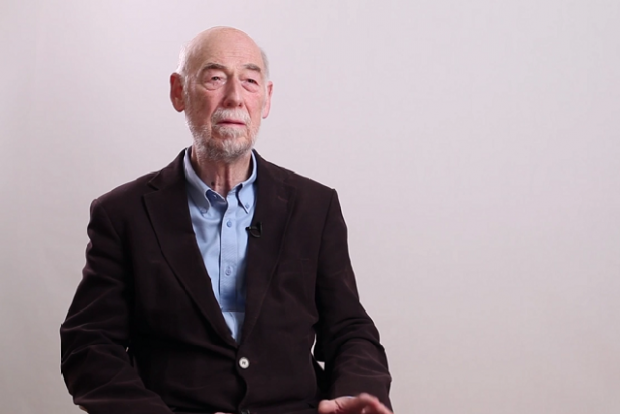# Logic and Mathematics

## Philosopher Denis Bonnay on Kant’s “Critique of Pure Reason”, logical inferences, and attempts to divide mathematics and logic

### videos | November 9, 2016

What is the basis for the debate between logic and mathematics? How did Kant contribute to this debate? Is it possible to prove mathematical truths based on logic alone? These and other questions are answered by Assistant Professor in the Department of Philosophy, Paris Ouest University, Denis Bonnay.

Logic and mathematics are two sister-disciplines, because logic is this very general theory of inference and reasoning, and inference and reasoning play a very big role in mathematics, because as mathematicians what we do is we prove theorems, and to do this we need to use logical principles and logical inferences.Historian and Philosopher of Logic Stephen Read on the history of paradoxes, semantic paradoxes, and its direct connection to the foundations of mathematics

If we just look at mathematical concepts, there is not enough in them to ground mathematical truths. He took a famous example: how do we know that 7+5=12? And if you look at the concept of 7 and if you look at the concept of 5, and if you look at the concept of a sum of two numbers, then nothing is going to tell you that the sum equals 12. So you will have to produce, to generate the truth, for example, by counting on your fingers, and that would rely on some sort of intuition.

The result of this was some sort of blurring of the lines between logic and mathematics, because in order to succeed we need to put axioms, principles in the logical systems which were not so logical anymore and which were a bit borderline themselves between logic and mathematics.Assistant Professor, Department of Philosophy, Paris Ouest University
Did you like it? Share it with your friends!
Published items
0779
To be published soon
+92

#### Most viewed

New